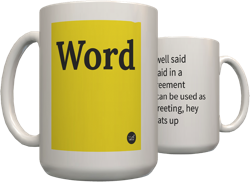Top definition
critical state soil theory:
A unified mathematical model of behaviour of saturated remoulded clay in triaxial compression tests if continuously distorted( sheared) untill it flow as a frictional fluid,will come to a well-defined critical state distorting under constant volume,v,constant mean effective stress,p1, and constant deviator stress,q, critical state line CSL) governed by

v=T-h ln p1
q=Mp1

where v= specific volume,1+e,where e is the voids ratio
T ,h and M are soil constants,

q= d1-d3,
p1=1/3(d1+2d3)

where d1,effective axial stress, d2=d3 are the the effective cell pressure,
effective pressure d1=(D1-u) ,where D1 is the Total axial stress and u the pore water pressure
similarly d2=(D2-u)=d3=(D3-u) in a triaxial test, d2=d3
The above thery is assumed to apply to undisturbed soils and other granular materials.

This theory is after Roscoe,Schofiled and Wroth (1968)
Critical state soil theory: applicable in earth dams, embankments,soft grounds, piles on soils and foundations on soils
by ccdc June 26, 2009Get a critical state soil theory mug for your Facebook friend Günter.